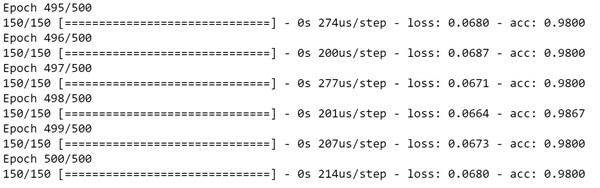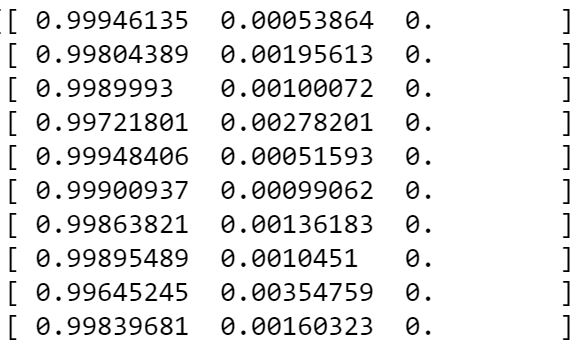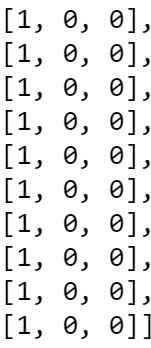Keras Multi-Class Classification Introduction

Introduction

Building neural networks is a complex endeavor with many parameters to tweak prior to achieving the final version of a model. On top of this, the two most widely used numerical platforms for deep learning and neural network machine learning models, TensorFlow and Theano, are too complex to allow for rapid prototyping. The Keras Deep Learning library for Python helps bridge the gap between prototyping speed and the utilization of the advanced numerical platforms for deep learning.Keras is a high-level API for building neural networks that runs on top of TensorFlow, Theano or CNTK. It allows for rapid prototyping, supports both recurrent and convolutional neural networks and runs on either your CPU or GPU for increased speed.

After reading this blog post you will be able to:

• Gain a better understanding of Keras

• Build a Multi-Layer Perceptron for Multi-Class Classification with Keras

Getting Started

We will build a 3 layer neural network that can classify the type of an iris plant from the commonly used Iris dataset. The Iris dataset contains three iris species with 50 samples each as well as 4 properties about each flower. Our neural network will take these 4 properties as inputs to try to predict which species the sample is from. This dataset contains 3 species, the Iris-setosa, Iris-versicolor and Iris-virginica.

First let’s import our data with the following python code.

#required library which holes the iris dataset
from sklearn.datasets import load_iris

#load the iris dataset
#our inputs will contain 4 features
X = iris.data[:, 0:4]
#the labels are the following
y = iris.target
#print the distinct y labels
print(np.unique(y))

The last line prints the distinct labels for each of the 3 species. You should be seeing these labels:  [0 1 2] In order for our classifier to work correctly we will first one hot encode our y vector by using the below code. To read more about one hot encoding I recommend my previous post One Hot Encoding with Pandas.

#One Hot Encode our Y:
from sklearn.preprocessing import LabelBinarizer
encoder = LabelBinarizer()
Y = encoder.fit_transform(y)

Building our Model

We will be now implementing a Multi-Layer Perceptron that contains 3 layers. Keras provides easy to use functionality to achieve this using its Sequential model. The Keras sequential model is a linear stack of layers. Keras provides different types of layers. We will be using the Dense layer type which is a fully connected layer that implements the operation output = activation(dot(input, kernel) + bias). To train our network we will be using the Stochastic Gradient Descent optimizer. You can read more about these and other Keras functionality in the Keras documentation.

Let’s start by importing our dependencies.

from keras.models import Sequential #Sequential Models
from keras.layers import Dense #Dense Fully Connected Layer Type
from keras.optimizers import SGD #Stochastic Gradient Descent Optimizer

We will now create our network architecture. As mentioned previously it will contain 3 layers.

• Our first layer will have 4 inputs corresponding to the 4 features we will be utilizing from the iris dataset.
• For our second layer (hidden layer) we will be using 5 neurons.
• Our third layer, will provide our classifications. This layer contains 3 neurons, corresponding to the 3 classes that we are aiming to predict.

Once we have our model built, we compiled our model. To compile our model we need to provide a loss function and an optimizer. The optimizer we defined to be the Stochastic Gradient Descent with a learning rate of 0.001, decay of 0.000001 and momentum of 0.9. Below is our function that returns this compiled neural network.

def create_network():
model = Sequential()

sgd = SGD(lr=0.001, decay=1e-6, momentum=0.9, nesterov=True)
model.compile(loss='categorical_crossentropy', optimizer=sgd, metrics=['accuracy'])
return model

Training our Model

To train our model we simply call the fit method of our model and provide the epochs and batch sizes we are using as below.

neural_network = create_network()
neural_network.fit(X,Y, epochs=500, batch_size=10)The fit method prints the accuracy and loss measured at each epoch. Our model is showing an accuracy of 98% at the 500th epoch. Keep in mind we haven’t split our data into test and training sets which you should be doing to get a better estimate of accuracy.

Making Predictions

With our trained model it’s easy to make predictions. First, we will make numpy print our probabilities in decimal form (removing the scientific notation). Then we will predict the first 10 samples in our X matrix.

import numpy as np
np.set_printoptions(suppress=True)

neural_network.predict(X[0:10], batch_size=32, verbose=0)

Our predicted class probabilities are:Our Actual classes from the first 10 samples Y[0:10] are:Conclusion

You should now be able to create a simple Multi-Layer Perceptron using the Keras library for deep learning. As shown above, this library allows rapid prototyping of neural networks allowing you to build models with few lines of codes.